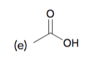# Problem: Draw the conjugate base for each of the following acids:

###### FREE Expert Solution
98% (405 ratings)
###### Problem Details

Draw the conjugate base for each of the following acids:What scientific concept do you need to know in order to solve this problem?

Our tutors have indicated that to solve this problem you will need to apply the Acid and Base Conjugates concept. If you need more Acid and Base Conjugates practice, you can also practice Acid and Base Conjugates practice problems.

What is the difficulty of this problem?

Our tutors rated the difficulty ofDraw the conjugate base for each of the following acids:...as low difficulty.

How long does this problem take to solve?

Our expert Organic tutor, Jonathan took 1 minute and 54 seconds to solve this problem. You can follow their steps in the video explanation above.

What textbook is this problem found in?

Our data indicates that this problem or a close variation was asked in Organic Chemistry - Klein 1st Edition. You can also practice Organic Chemistry - Klein 1st Edition practice problems.